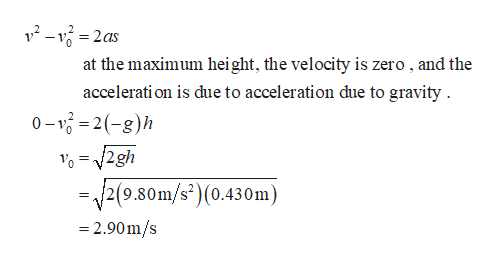# In this problem, you will apply kinematic equations to a jumping flea. Take the magnitude of free-fall acceleration to be 9.80 m/s2 . Ignore air resistance. A flea jumps straight up to a maximum height of 0.430 m . What is its initial velocity v0 as it leaves the ground?

Question
1771 views

In this problem, you will apply kinematic equations to a jumping flea. Take the magnitude of free-fall acceleration to be 9.80 m/s2 . Ignore air resistance. A flea jumps straight up to a maximum height of 0.430 m . What is its initial velocity v0 as it leaves the ground?

check_circle

Step 1

Write the kinematic equation, and s...help_outlineImage Transcriptionclosev-v2as at the maximum height, the velocity is zero , and the accelerati on is due to acceleration due to gravity 0-122(-g)h 0 2gh -/2(9.80m/s")(0.430m) = = 2.90m/s fullscreen

### Want to see the full answer?

See Solution

#### Want to see this answer and more?

Solutions are written by subject experts who are available 24/7. Questions are typically answered within 1 hour.*

See Solution
*Response times may vary by subject and question.
Tagged in

### Physics